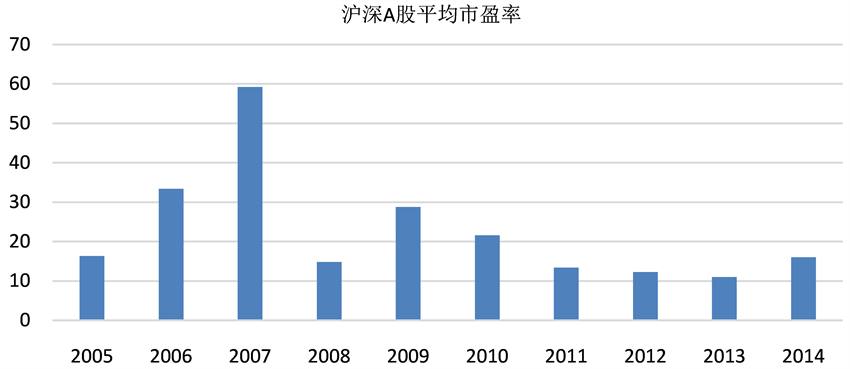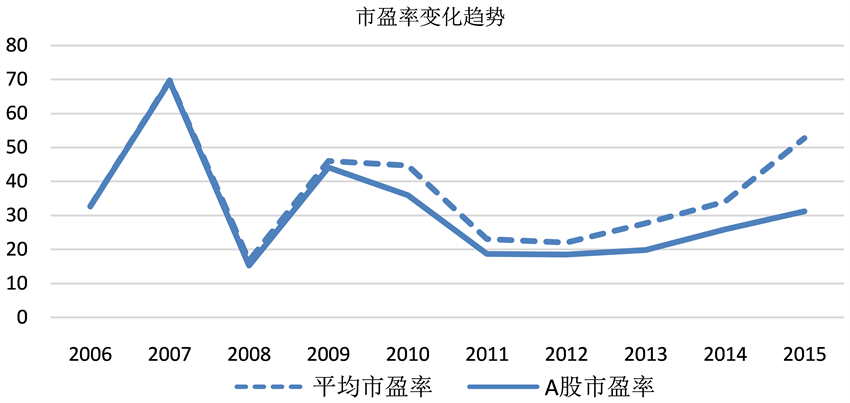股票市盈率影响因素实证分析 Empirical Analysis on the Influencing Factors of Stock’s P/E

• 全文下载: PDF(543KB)    PP.169-178   DOI: 10.12677/FIN.2018.84020
• 下载量: 339  浏览量: 618   科研立项经费支持

As an important index to evaluate the value of stock investment, the price earnings ratio is often used in the evaluation of the stock value of the company to judge the price of the stock, the size of the risk and the forecast of the future profitability of the company. So the specific factors of the price earnings ratio are analyzed, which are of great significance to realize the value of the price earnings ratio. Using the method of simple random sampling, this paper selects 34 stocks of the 180 index stock of SSE as the research object, uses the statistical method of regression analysis and quantitative analysis by adopting the processing tools of the table and the related software, in order to study the relationship between the factors in detail and the statistics of the factors. The data are analyzed by multiple linear regression using OLS and three effective total regression models and regression models are established. The validity of the regression model is explained from the fitting degree of each model and the contribution of the selected key factors to the price earnings ratio is confirmed, and the average P/E ratio and earnings per share are increased, which have the greatest impact on the P/E ratio. At the same time, investors can make use of these three effective regression models to reasonably predict the price earnings ratio of the stock, so as to make a more rational judgment of the investment value of the stock.

1. 引言及文献综述

2. 研究设计

2.1. 研究方法

$\mathrm{min}\sum {e}_{i}^{2}=\mathrm{min}\sum {\left({Y}_{i}-{\stackrel{^}{Y}}_{i}\right)}^{2}=\mathrm{min}\sum {\left({Y}_{i}-{\stackrel{^}{\beta }}_{1}-{\stackrel{^}{\beta }}_{2}{X}_{2i}-\cdots -{\stackrel{^}{\beta }}_{k}{X}_{ki}\right)}^{2};i=1,2,\cdots ,n$

$\frac{\partial \left(\sum {e}_{i}^{2}\right)}{\partial {\stackrel{^}{\beta }}_{j}}=0;\left(j=1,2,\cdots ,k\right)$

$-2\sum \left({Y}_{i}-{\stackrel{^}{\beta }}_{1}-{\stackrel{^}{\beta }}_{2}{X}_{2i}-\cdots -{\stackrel{^}{\beta }}_{k}{X}_{ki}\right)=0$ (1)

$-2\sum \left({Y}_{i}-{\stackrel{^}{\beta }}_{1}-{\stackrel{^}{\beta }}_{2}{X}_{2i}-\cdots -{\stackrel{^}{\beta }}_{k}{X}_{ki}\right){X}_{2i}=0$ (2)

$\cdots$

$-2\sum \left({Y}_{i}-{\stackrel{^}{\beta }}_{1}-{\stackrel{^}{\beta }}_{2}{X}_{2i}-\cdots -{\stackrel{^}{\beta }}_{k}{X}_{ki}\right){X}_{ki}=0$ (3)

$\left[\begin{array}{c}\sum {e}_{i}\\ \sum {e}_{i}{X}_{2i}\\ ⋮\\ \sum {e}_{i}{X}_{ki}\end{array}\right]=\left[\begin{array}{c}0\\ 0\\ ⋮\\ 0\end{array}\right]$ (4)

$\left[\begin{array}{c}\sum {e}_{i}\\ \sum {e}_{i}{X}_{2i}\\ ⋮\\ \sum {e}_{i}{X}_{ki}\end{array}\right]=\left[\begin{array}{cccc}1& 1& \cdots & 1\\ {X}_{21}& {X}_{22}& \text{​}\cdots & {X}_{2n}\\ ⋮& ⋮& & ⋮\\ {X}_{k1}& {X}_{k2}& \cdots & {X}_{kn}\end{array}\right]\left[\begin{array}{c}{e}_{1}\\ {e}_{2}\\ ⋮\\ {e}_{n}\end{array}\right]={X}^{\text{T}}e=\left[\begin{array}{c}0\\ 0\\ ⋮\\ 0\end{array}\right]$ (5)

2.2. 数据说明和研究变量的选取

2.3. 实证模型Table 1. Variable definition and calculation formula

$Y={\alpha }_{0}+\underset{i=1}{\overset{8}{\sum }}{\alpha }_{i}{X}_{i}+\epsilon$ (6)

$Y={\beta }_{0}+{\beta }_{4}{X}_{4}+{\beta }_{6}{X}_{6}+{\beta }_{7}{X}_{7}+\eta$ (7)

$Y={\varphi }_{0}+{\varphi }_{6}{X}_{6}+{\varphi }_{7}{X}_{7}+\mu$ (8)

3. 沪深股市股票市盈率影响因素实证检验

3.1. 中国沪深股市总体市盈率分析Table 2. P/E ratio of A-share main board in May 2016

3.2. 中国沪深股市市盈率波动分析Figure 1. Average P/E ratio of Shanghai A-share in 2005-2014Figure 2. Average P/E ratio of Shenzhen stock market and the change trend of P/E Ratio A-Share main board in 2006-2015

3.3. 模型的回归结果分析

3.3.1. 模型I的回归结果分析

$Y={\alpha }_{0}+\underset{i=1}{\overset{8}{\sum }}{\alpha }_{i}{X}_{i}+\epsilon$ (9)

$\begin{array}{l}\stackrel{^}{Y}=65.57-0.28{X}_{1}+0.056{X}_{2}-1.13{X}_{3}+0.04{X}_{4}-0.34{X}_{5}+1.06{X}_{6}+0.36{X}_{7}+5.44{X}_{8}\\ \left(26.18\right)\left(0.19\right)\left(0.54\right)\left(0.63\right)\left(0.29\right)\left(0.59\right)\left(0.66\right)\left(0.62\right)\left(18.18\right)\\ t=\text{}\left(2.50\right)\left(-1.45\right)\left(0.10\right)\left(-1.79\right)\left(2.15\right)\left(-0.57\right)\left(3.59\right)\left(2.09\right)\left(0.299\right)\end{array}$

1) 根据回归系数的正负，可以判断Y与 ${X}_{i},i=1,2,\cdots ,8$ 间是正相关还是负相关，即回归系数为正，它们间就是正相关，回归系数为负，它们间就是负相关。在0.05的显著性水平下，一般情况下，t值大于2，P值小于0.05，就说明解释变量对被解释变量具有显著性影响。

Y与 ${X}_{1}$ 经证明具有弱反向关系，因为 ${X}_{1}$ 的回归系数为−0.28，且在0.05的显著性水平下，t值和P值没有通过假设检验。

2) Y与 ${X}_{2}$ 具有正向关系，因为 ${X}_{2}$ 的回归系数为0.056，且在0.05的显著性水平下，t值和P值没有通过假设检验，因此，可以认为Y与 ${X}_{2}$ 之间不存在相关关系。Table 3. Multivariate regression estimation results of model I

3) Y与 ${X}_{3}$ 之间的关系经证实具有弱反向关系，因为 ${X}_{3}$ 的回归系数为−1.12，且在0.05的显著性水平下，t值和P值没有通过假设检验。

4) Y与 ${X}_{5}$ 具有反向关系，因为 ${X}_{5}$ 的回归系数为−0.33，而且在0.05的显著性水平下，t值和P值检验是通过假设检验的。

5) Y与 ${X}_{8}$ 之间的关系经证实具有弱正相关关系，因为 ${X}_{8}$ 的回归系数为−5.44，且在0.05的显著性水平下，t值和P值没有通过假设检验。

3.3.2. 模型II的回归结果分析

$Y={\beta }_{0}+{\beta }_{4}{X}_{4}+{\beta }_{6}{X}_{6}+{\beta }_{7}{X}_{7}+\eta$ (10)

$\begin{array}{l}\stackrel{^}{Y}=23.792+0.035{X}_{4}+0.313{X}_{6}+0.349{X}_{7}\\ \left(12.39\right)\left(0.27\right)\left(0.51\right)\text{}\left(0.11\right)\\ t=\text{}\left(1.92\right)\left(0.13\right)\left(2.61\right)\text{}\left(3.28\right)\end{array}$

3.3.3. 模型III的回归结果分析

$Y={\varphi }_{0}+{\varphi }_{6}{X}_{6}+{\varphi }_{7}{X}_{7}+\mu$ (11)Table 4. Multivariate regression estimation results of model IITable 5. Multivariate regression estimation results of model III

$\begin{array}{l}\stackrel{^}{Y}=24.802+0.299{X}_{6}+0.345{X}_{7}\\ \left(\text{9}\text{.56}\right)\left(\text{0}\text{.49}\right)\text{}\left(\text{0}\text{.10}\right)\\ t=\text{}\left(2.596\right)\text{}\left(2.61\right)\text{}\left(3.48\right)\end{array}$

3.3.4. 模型回归结果总体分析

4. 研究结论

1) 不同的指标对市盈率的贡献度是有差异的。从回归分析结果来看，资产负债率、净利润增长率、净资产收益率、每股净资产、流通股比例这些指标对市价盈利比率的解释作用不明显，无论是在总体回归模型还是在分回归模型中，行业平均市盈率和每股收益增长率两个因素对市价盈利比率的影响程度和解释能力都比较高。

2) 模型从实证方面证明了它达到了预期的结果，广大投资者可使用模型来推算某只股票的市盈率。在使用回归模型时，广大投资者可依据自己了解的行业状况来选取恰当的模型，但无论选取这3个模型中的哪一个模型，投资者都应把重心放在行业平均市盈率、每股收益增长率两个指标上。

3) 在合理运用的前提下，市盈率可很好地应用于投资决策，多元回归模型分析结果的误差率就很好地证实了这一点。所以，广大投资者在决策时可依据估算的市盈率知道哪只股票可以购买或者持有，从而为自己实现资产价值增值做出理性的计划，而不是毫无目的的进行投资。

  陈英. 我国上市公司市盈率影响因素的实证研究[C]. 杭州: 浙江大学, 2003: 15-17.  Graham, B. and Dodd, D. 证券分析[M]. 海南: 海南出版社, 1999: 474-484.  Loughlin, J.J. (1996) Determinants of the Price-Earnings Multiple for the Standard & Poors 500 Composite Stock Index and the Effects of Determinants Volatility. Ph.D. Dis-sertation, Saint Louis University, St. Louis, 66-70.  Whibeck, K. (1989) Accounting Measurement Price-Earnings Ratio and the Information Content of Security Prices. Journal of Accounting Research, 27, 111-152. https://doi.org/10.2307/2491068  Fuller, R.J., et al. (1992) It’s Not Highly-Piggledy Growth. The Journal of Por-folio Management, 18, 38-45. https://doi.org/10.3905/jpm.1992.409400  Penman, S.H. and Sougiannis, T. (1997) The Dividend Displacement Property and the Substitution of Anticipated Earnings for Dividends in Equity Valuation. Accounting Review, 16, 78-88.  李社环, 林琼慧. 公司合理的负债比率与股票市盈率的关系[J]. 预测, 1998, 17(6): 60-62.  何治国. 中国股市风险因素实证研究[J]. 经济评论, 2001, 21(3): 81-84.  曾晓洁, 何小锋, 晏青. 市盈率隐含的公司业绩增长潜力一中国股市市盈率实证分析[J]. 经济科学, 2002, 23(3): 50-61.  白娜, 顾卫俊. 上证30指数股市盈率实证分析[J]. 浙江大学学报(人文社会科学版), 2002, 32(2): 149-156.  庞皓. 计量经济学[M]. 北京: 科学出版社, 2014: 63-81.  吴明礼. 我国股市市盈率结构分布和分析[J]. 数量经济技术研究, 2001, 17(5): 99-102.